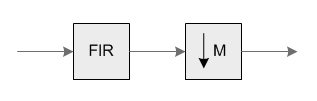Project Ne10 An open, optimized software library for the ARM architecture.
Finite Impulse Response (FIR) Decimation

## Functions

ne10_result_t ne10_fir_decimate_init_float (ne10_fir_decimate_instance_f32_t *S, ne10_uint16_t numTaps, ne10_uint8_t M, ne10_float32_t *pCoeffs, ne10_float32_t *pState, ne10_uint32_t blockSize)
Initialization function for the floating-point FIR decimator. More...

void ne10_fir_decimate_float_c (const ne10_fir_decimate_instance_f32_t *S, ne10_float32_t *pSrc, ne10_float32_t *pDst, ne10_uint32_t blockSize)
Specific implementation of ne10_fir_decimate_float using plain C. More...

void ne10_fir_decimate_float_neon (const ne10_fir_decimate_instance_f32_t *S, ne10_float32_t *pSrc, ne10_float32_t *pDst, ne10_uint32_t blockSize) asm("ne10_fir_decimate_float_neon")
Specific implementation of ne10_fir_decimate_float using NEON SIMD capabilities. More...

## Variables

void(* ne10_fir_decimate_float )(const ne10_fir_decimate_instance_f32_t *S, ne10_float32_t *pSrc, ne10_float32_t *pDst, ne10_uint32_t blockSize)
Processing function for the floating-point FIR decimator. More...

## Detailed Description

These functions combine an FIR filter together with a decimator. They are used in multirate systems for reducing the sample rate of a signal without introducing aliasing distortion. Conceptually, the functions are equivalent to the block diagram below:Components included in the FIR Decimator functions
When decimating by a factor of `M`, the signal should be prefiltered by a lowpass filter with a normalized cutoff frequency of `1/M` in order to prevent aliasing distortion. The user of the function is responsible for providing the filter coefficients.

The FIR decimator functions provided in the CMSIS DSP Library combine the FIR filter and the decimator in an efficient manner. Instead of calculating all of the FIR filter outputs and discarding `M-1` out of every `M`, only the samples output by the decimator are computed. The functions operate on blocks of input and output data. `pSrc` points to an array of `blockSize` input values and `pDst` points to an array of `blockSize/M` output values. In order to have an integer number of output samples `blockSize` must always be a multiple of the decimation factor `M`.

The library provides functions for floating-point data types.

Algorithm:
The FIR portion of the algorithm uses the standard form filter:
```     y[n] = b * x[n] + b * x[n-1] + b * x[n-2] + ...+ b[numTaps-1] * x[n-numTaps+1]
```
where, `b[n]` are the filter coefficients.
The `pCoeffs` points to a coefficient array of size `numTaps`. Coefficients are stored in time reversed order.
```     {b[numTaps-1], b[numTaps-2], b[N-2], ..., b, b}
```
`pState` points to a state array of size `numTaps + blockSize - 1`. Samples in the state buffer are stored in the order:
```     {x[n-numTaps+1], x[n-numTaps], x[n-numTaps-1], x[n-numTaps-2]....x, x, ..., x[blockSize-1]}
```
The state variables are updated after each block of data is processed, the coefficients are untouched.
Instance Structure
The coefficients and state variables for a filter are stored together in an instance data structure. A separate instance structure must be defined for each filter. Coefficient arrays may be shared among several instances while state variable array should be allocated separately. There are separate instance structure declarations for each of the 3 supported data types.
Initialization Functions
There is also an associated initialization function for each data type. The initialization function performs the following operations:
• Sets the values of the internal structure fields.
• Zeros out the values in the state buffer.
• Checks to make sure that the size of the input is a multiple of the decimation factor.
Use of the initialization function is optional. However, if the initialization function is used, then the instance structure cannot be placed into a const data section. To place an instance structure into a const data section, the instance structure must be manually initialized. The code below statically initializes each of the 3 different data type filter instance structures
```*ne10_fir_decimate_instance_f32_t S = {M, numTaps, pCoeffs, pState};
```
where `M` is the decimation factor; `numTaps` is the number of filter coefficients in the filter; `pCoeffs` is the address of the coefficient buffer; `pState` is the address of the state buffer. Be sure to set the values in the state buffer to zeros when doing static initialization.
Fixed-Point Behavior
Care must be taken when using the fixed-point versions of the FIR decimate filter functions. In particular, the overflow and saturation behavior of the accumulator used in each function must be considered. Refer to the function specific documentation below for usage guidelines.

## Function Documentation

 void ne10_fir_decimate_float_c ( const ne10_fir_decimate_instance_f32_t * S, ne10_float32_t * pSrc, ne10_float32_t * pDst, ne10_uint32_t blockSize )

Specific implementation of ne10_fir_decimate_float using plain C.

Definition at line 429 of file NE10_fir.c.

 void ne10_fir_decimate_float_neon ( const ne10_fir_decimate_instance_f32_t * S, ne10_float32_t * pSrc, ne10_float32_t * pDst, ne10_uint32_t blockSize )

Specific implementation of ne10_fir_decimate_float using NEON SIMD capabilities.

 ne10_result_t ne10_fir_decimate_init_float ( ne10_fir_decimate_instance_f32_t * S, ne10_uint16_t numTaps, ne10_uint8_t M, ne10_float32_t * pCoeffs, ne10_float32_t * pState, ne10_uint32_t blockSize )

Initialization function for the floating-point FIR decimator.

Parameters
 [in,out] *S points to an instance of the floating-point FIR decimator structure. [in] numTaps number of coefficients in the filter. [in] M decimation factor. [in] *pCoeffs points to the filter coefficients. [in] *pState points to the state buffer. [in] blockSize number of input samples to process per call.
Returns
The function returns NE10_OK if initialization was successful or NE10_ERR if `blockSize` is not a multiple of `M`.

Description:

`pCoeffs` points to the array of filter coefficients stored in time reversed order:
```   {b[numTaps-1], b[numTaps-2], b[N-2], ..., b, b}
```
`pState` points to the array of state variables. `pState` is of length `numTaps+blockSize-1` words where `blockSize` is the number of input samples passed to `arm_fir_decimate_f32()`. `M` is the decimation factor.

Definition at line 98 of file NE10_fir_init.c.

## Variable Documentation

 void(* ne10_fir_decimate_float) (const ne10_fir_decimate_instance_f32_t *S, ne10_float32_t *pSrc, ne10_float32_t *pDst, ne10_uint32_t blockSize)

Processing function for the floating-point FIR decimator.

Parameters
 [in] *S points to an instance of the floating-point FIR decimator structure. [in] *pSrc points to the block of input data. [out] *pDst points to the block of output data. [in] blockSize number of input samples to process per call.

Definition at line 168 of file NE10_init_dsp.c.